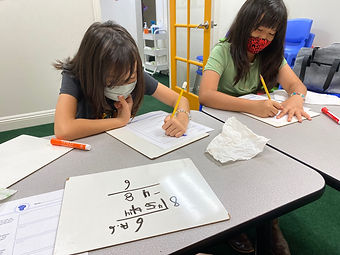## Ms. Dawn

### Target 1​

###### Lesson Type:

Continuation

Number Operation

:

Number Reltionships

Compare numbers, recording the results of the comparison with the symbol >, =, and <.

###### 1:

Compare decimals (up to the hundredths place).

###### 2:

When comparing decimals, understand to look at the greatest place-value first.

###### 3:

Explain how a number could have a lesser value, even if it has more digits than another (i.e., 4.33<5.2).

4th

###### Vocabulary:

Place value, Tenths, Hundredths, Decimal, "And," Compare, Explain

Activities:

1. Students rolled a decimal die and were comparing two different decimals addressing greater than, less than, or equal to. After a few times of verbally saying it outloud, they filled out a comparison sheet. They needed to say which one was greater than, draw a picture that represented the comparison and finally explain their thinking of why one decimal number was greater than the other. Students needed to use the correct vocabulary (place value) to address their explanation.

2. Students were given a place value chart and die. They were told what place value to go up to and roll their die for each place value block. After rolling a number, students read their created decimal outloud using the correct vocabulary. After each student read it outloud, they compared it with their peers.### Home Exploration

###### Guiding Questions:## Absent Students:

### Target 2

:

###### 1:

Identify the correct arithmetic processes based on the information presented in word problems.

###### 2:

Find the key words in word problems that indicate the correct arithmetic process to use: Addition: add, altogether, both, combined, in all, plus, sum, total; Subtraction: difference, fewer, how many/much more, left, less, minus, remains; Multiplication: times, product, each, twice; and Division: how many in each, divided by, quotient, goes into, split evenly.

###### 3:

Find and use the needed information in a word problem to solve.

4th

###### Vocabulary:

CUBES, Operation, Table, Arithmetic

Activities:

1. Students answered word problems using strategies they've learned from previous classes. For example, "CUBES" method to help them break down the problem. Also, different methods for multiplication when they needed to.

2. Students needed to create tables to solve their word problems along with a variety of operations.### Home Exploration

###### Guiding Questions:### Target 3

:

###### 1:

Understand the role of place-value in division strategies.

###### 2:

Use the standard algorithm for solving multi-digit division equations.

###### 3:

Understand that there can be a remainder in a division problem.

4th

###### Vocabulary:

Divide, Remainder

Activities:

1. Students solved a variety of division word problems that ended with remainders. They needed to figure out what to do with the remainder based on the question asked. They either needed to included the remainder in their answer or leave it out.### Home Exploration